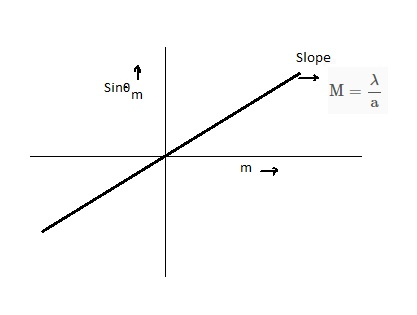# Suppose you observe a series diffraction minima and plot sin ? m vs. for those minima. What...

## Question:

Suppose you observe a series diffraction minima and plot {eq}\sin \theta_m{/eq} vs. for those minima. What type of graph would you expect get? Describe not only the shape o the graph but also the parameters that would describe it.

## Constructive and destructive Interference:

When the light passes any slit, then the redistribution of the light takes place, this superposition of the light waves is known as interference. If the waves are in phase during superposition, then it is known as constructive interference.The Path difference between the waves is given by the following expression

{eq}\rm \delta x = a\sin\theta_{m} {/eq}

where

• a is the slit width
• {eq}\rm \theta_{m} {/eq} is the angular position of the fringe

When the path difference is equal to the integral multiple of the wavelength, then the constructive interference or maxima forms, therefore

{eq}\begin{align} \rm \delta x &= \rm m \lambda \\ \rm a\sin\theta_{m} &= \rm m \lambda \\ \sin\theta &= \rm \dfrac{\lambda}{a} m \\ \end{align} {/eq}

where

• m is the order of the fringe
• {eq}\rm \lambda {/eq} is the wavelength

For the plot of the {eq}\rm \sin\theta_{m} {/eq} vs {eq}\rm m {/eq}

the above equation resembles with the equation of the straight line passing through the origin i.e. {eq}\rm y = Mx {/eq}

where "M" represents the slope of the graph which would be equal to, {eq}\rm M = \dfrac{\lambda}{a} {/eq}